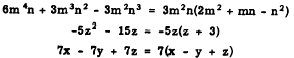Chapter 10 - Factoring PolynomialsCustom SearchCHAPTER 10 FACTORING POLYNOMIALS A factor of a quantity N, as defined in chapter 2 of this course, is any expression which can be divided into N without producing a remainder. Thus 2 and 3 are factors of 6, and the factors of 5x are 5 and x. Conversely, when all of the factors of N are multiplied together, the product is N. This definition is extended to include polynomials. The factors of a polynomial are two or more expressions which, when multiplied together, give the polynomial as a product. For example, 3, x, and x2 - 4 are factors of 3x3 - 12x, as the following equation shows: (3)(x)(x2 - 4) = 3x3 - 12x The factors 3 and x, which are common to both terms of the polynomial 3x3 - 12x, are called COMMON FACTORS. The distributive principle, mentioned in chapters 3 and 9 of this course, is an important part of the concept of factoring. It may be stated as follows: If the sum of two or more quantities is multiplied by a third quantity, the. product is found by applying the multiplier to each of the original quantities separately and summing the resulting expressions. It is this principle which allows us to separate common factors from the terms of a polynomial. Just as with numbers, an algebraic expression is a prime factor if it has no other factors except itself and 1. The factor x2 - 4 is not prime, since it can be separated into x - 2 and x + 2. The factors x -2 and x+2 are both prime factors, rime they cannot be separated into other factors. The process of finding the factors of a polynomial is called FACTORING. An expression is said to be factored completely when it has been separated into its prime factors. The polynomial 3x3 - 12x is factored completely as follows: 3x3 - 12x = 3x(x - 2)(x + 2) COMMON FACTORS Factoring any polynomial begins with the removal of common factors. Notice that "removal" of a factor does not mean discarding it. To remove a factor is to insert parentheses and move the factor outside the parentheses as a common multiplier. The removal of common factors proceeds as follows: 1. Inspect the polynomial and find the factors which are common to all terms. These common factors, multiplied together, comprise the ‘largest common factor ." 2. Mentally divide each term of the polynomial by the largest common factor and write the quotients within a set of parentheses. 3. Write the largest common factor outside the parentheses as a common multiplier. For example, the expression x2y - xy2 contains xy as a factor of each term. Therefore, it is factored as follows: x2y - xy2 = xy(x - Y) Other examples of factoring by the removal of common factors are found in the following expressions:In selecting common factors, always remove as many factors as possible from each term in order to factor completely. For example, x is a factor of 3ax2 - 3ax, so that 3ax2 - 3ax is equal to x(3ax -3a). However, 3 and a are also factors. Thus the largest common factor is 3ax. When factored completely, the expression is as follows: 3ax2 - 3ax = 3ax(x - 1) Practice problems: Remove the common factors:LITERAL EXPONENTS It is frequently necessary to remove common factors involving literal exponents; that is, exponents composed of letters rather than numbers. A typical expression involving literal exponents is x2a + xa , ’ in which xa is a common factor. The factored form is xa(xa + 1). Another example of this type is am+n, + 2am. Remember that (am·n) is equivalent to (am)(an). Thus the factored form is as follows:Integrated Publishing, Inc. - A (SDVOSB) Service Disabled Veteran Owned Small Business